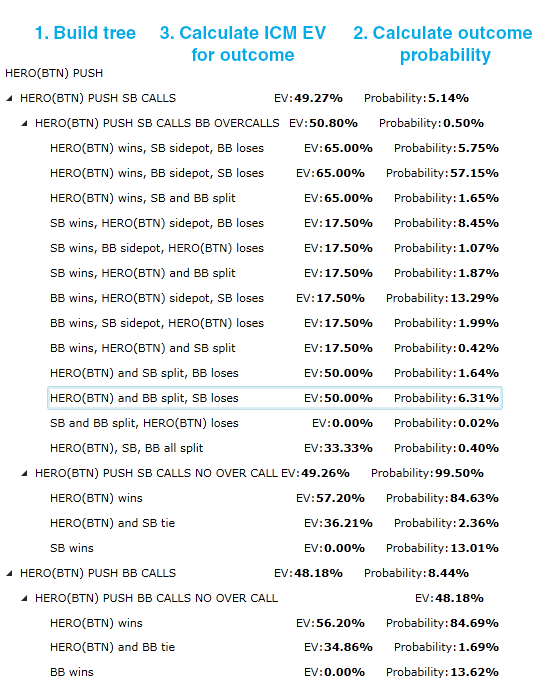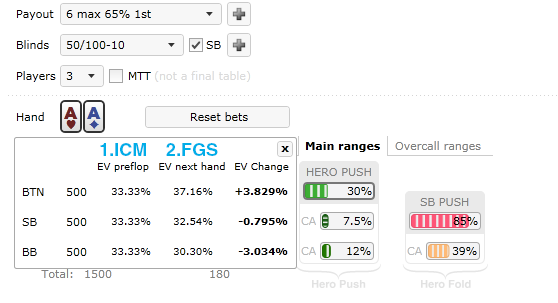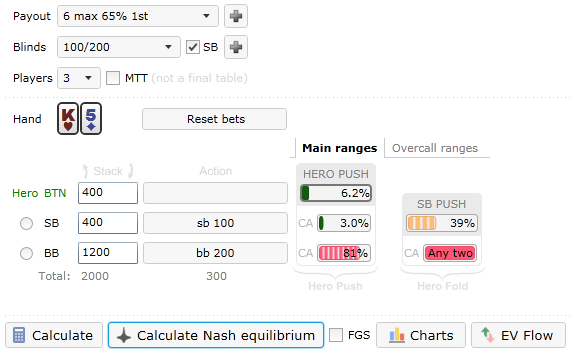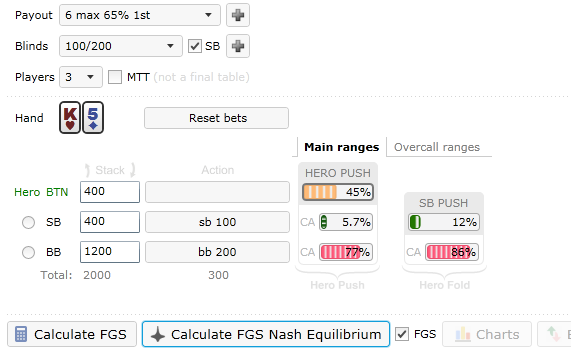BLACK FRIDAY SALE ENDS IN
-167 days
:
0 hours
:
-29 minutes
:
-23 seconds
HomeBlog

# FGS (Future Game Simulations) Calculations Explained

## 20 February 2014 by Q.

The FGS (Future Game Simulations) model is based on the ICM model, and its goal is to lift some well-known ICM limitations.

The ICM limitations, which FGS takes care of, are:

• ICM ignores the sizes of the big blind, small blind and ante.
• ICM ignores the position of players in relation to the blinds.
• ICM ignores the fact that the blinds are moving around the table.

Warning: FGS is much more complex than usual ICM! It usually takes a reasonable amount of time for poker players to understand basic ICM. FGS will require even more effort.

In this article we will see how FGS works, in what ways exactly it is based on ICM, where it works similar to ICM, and finally, what makes it very different.

First, let’s revisit how usual ICM works.

## How usual ICM calculation works

In this simple example we calculate our ICM EV of open pushing aces from the button.

1. We build a tree of potential outcomes after our push and calculate the probability of each outcome.2. The next step is calculating the probability of each outcome. To calculate the probability that SB and BB will Fold, Call or Overcall, we take into account the card removal effect, and then apply some combinatory formulas. This is relatively simple: we just get certain chances that if we push, then a) SB will call x% of the time, b) y% of the time BB will overcall when he/she calls, and c) z% of the time everyone will fold, etc.

Once we know those probabilities, we need to find out how each outcome that involves a showdown plays out postflop. In 2-way confrontations there are 3 potential outcomes, and in 3-way confrontations there are 13 potential outcomes. We need calculate the probability of each outcome.

3. Once we know the probability of each outcome, we need to calculate ICM EV of Hero stack for each outcome. With ICM this is pretty straightforward: we just take our payout and the stacks that are the result of this specific outcome (say Hero wins the pot, and SB and BB split their part of the side pot), and get the resulting EV for hero.

Example: If we end up with 0 chips in any outcome (because we lose to some bigger or equal stack) our EV in that outcome is 0% of prize pool, if we aren’t in the money already.

Once steps 1, 2 and 3 are done we can get our final result of Hero Push EV.

The probabilities of each outcome and Hero ICM EV for each outcome is all that’s required for Push EV calculation. We just multiply the probability of each outcome by ICM EV of that outcome, and then add those products to get the total ICM EV of our Push action.

In other words, Push EV is a weighted sum of each potential outcome EV. The weight here is the actual probability of each outcome, so the less probable it is, the smaller weight it has.

## How FGS works compared to ICM

In FGS we compute the probability of each outcome the same way as we do in usual ICM calculation. Steps 1 and 2 are the same.

Where things get really interesting with FGS isat the final 3rd step, which involves the calculation of EV of the outcome. To account for all the blinds and positions, we have to do some smart things before we can get our FGS equity result.

In Future Game Simulation the 3rd step looks as follows:

1. Take the resulting stacks of the outcome ordered by position.2. Rotate the resulting stacks according to the positions, meaning that now Hero stack is the same, but it is in the position where Hero will be in the next hand.
3. We take blinds into account and perform the Nash equilibrium ranges calculation for this new rotated spot.
4. Based on calculated ranges we calculate how Hero EV will look after the next hand IF it were played according to this Nash equilibrium strategy. In ICMIZER this process is called EV Flow calculation.
5. This value for Hero stack after the next hand is the FGS equity value for the outcome we are currently working with.

We do this for each potential outcome. Because of this we need to do a lot of expensive additional calculations in order to get FGS value. The most expensive part here is the Nash equilibrium calculation, but calculating how EV will look after the hand where players will push according to calculated Nash ranges also takes some time.

## Demonstration of difference in ICM and FGS equity calculations

We are 3 handed in a typical 6-max poker tournament.

Let’s say that in our example hand Hero is UTG, and we are analyzing EV of a specific outcome where hero will be on the big blind position and all stacks will be equal.

Since ICM doesn’t care about blinds or positions, according to ICM our stack will have the same equity as everyone else, 33.33% of the prize pool.

We can see that value under 1.ICM on the picture above.

However, when we calculate Nash ranges for this hand, and we calculate the EV flow or how each player’s EV will look after this hand, we can see that the equity of the player on the big blind position is far worse than the equity of the player on the button.

The second column would give us the FGS value of the BB stack for this spot. Since it has lower equity than ICM value, it means that from FGS perspective this outcome is less desirable than if we end up on SB or BTN position.

This effect will lead to a much wider pushing range for BTN, compared to ICM. It makes common sense as well: as players we know that posting a blind next hand when stacks are shallow is a good motivator to be wider than we normally would.

Without FGS we do this by making a so-called “negative EV” ICM based push. We adjust min Diff EV to some negative value.

With FGS we don’t need to think about the size of negative min diff EV value, instead we make a +EV FGS equity based push!

To showcase some concrete benefits of the FGS model, here's a real-world example.

## A real-world example of FGS calculation and comparison to ICM calculation

We will examine the following situation.

### Nash equilibrium pushing ranges according to usual ICMHere we are under the gun with 2 blinds and we have performed usual ICM based Nash equilibrium calculations.

We can see the problems with ICM: UTG range is so tight because it assumes that SB will be pushing wide. Of course, if that was the case we would be more likely to fold and dream about cashing in if BB busted SB.

However, everybody realizes that next hand we are the big blind and SB will blind out after us, meaning he/she is very unlikely to push wide here, as single-hand-aware ICM calculation suggests. We would be trying to make a negative shove since with 2 blinds it makes sense that we have to be pushing at least 30% of hands here, and probably more (if we think about common sense and the next hand).

Now we look at FGS-based ranges:

### Nash equilibrium pushing ranges according to FGSWe can see that FGS took into account what we know from our game experience! SB isn't pushing 39% as he/she did before. If we fold, he/she would be only playing 12% of hands, limiting himself to nuts and waiting for us to bust.

Our range has also changed, we are now up from 6% to 45%, and basically pushing half of the hands we are dealt.

It should be pretty obvious that the player who adopts FGS-based ranges on SB and UTG positions in this spot would be making much better decisions and winning more money than the player who follows traditional ICM-based advice.

The curious thing about this example is that UTG push range literally swapped with SB push range after future games were taken into account.

## Conclusion

FGS is not a “silver bullet”. Of course the FGS model is not perfect and also has its limitations. The most obvious limitation is our assumption that all players will play according to Nash in the upcoming hands. This is often not the case, and the actual behavior in the next hand would have a reasonable impact on our decision in the current hand.

If we want to go beyond basic ICM and take the future into account, we have to make some assumptions. Assuming that players will push according to Nash equilibrium—and at the highest level of play, that’s how players tend to behave— is a fair way to estimate the price of posting the big blind. This is only a minor shortcoming compared to the benefits FGS provides.

We can see that the FGS model takes the best from the ICM model, but allows for more accurate ranges in tournament hands where players are relatively short and posting big blind has a serious impact on the player’s equity and future prospects in the tournament.